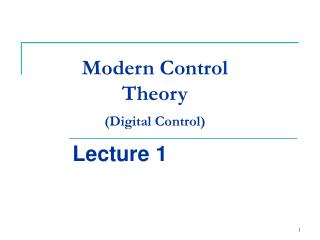# Modern Control Theory (Digital Control) - PowerPoint PPT PresentationDownload PresentationModern Control Theory (Digital Control)

Modern Control Theory (Digital Control)Download Presentation## Modern Control Theory (Digital Control)

- - - - - - - - - - - - - - - - - - - - - - - - - - - E N D - - - - - - - - - - - - - - - - - - - - - - - - - - -
##### Presentation Transcript

1. Course Overview • Analog and digital control systems • MM 1 – introduction, discrete systems, sampling. • MM 2 – discrete systems, specifications, frequency response methods. • MM 3 – discrete equivalents, design by emulation. • MM 4 – root locus design. • MM 5 – root locus design.

2. Outline • Short repetition of analog control methods • Introduction to digital control • Digitization • Effect of sampling • Sampling • Spectrum of a sampled signals • Sampling theorem • Discrete Systems • Z-transform • Transfer function • Pulse response • Stability

3. Digitization • Analog Control System For example, PID control continuous controller r(t) e(t) ctrl. filter D(s) u(t) plant G(s) y(t) + - sensor H(s)

4. System caracteristics • Transfer function • Characteristic equation • 1+D(s)G(s)H(s) = 0 • Poles are the roots of the characteristic equation

5. Time functions associated with poles

6. Second-order system • Transfer function • is the damping ratio • is the undamped natural frequency

7. Rise time, overshoot and settling time

8. Response og second-order system versus

9. Bode-plot design • Determin the open loop gain end phase as function of • Evaluate the phase margin and gain margin • Adjust the margins by use of poles, zeros and gain scheduling.

10. Bode plot

11. Digitization • Analog Control System For example, PID control continuous controller r(t) e(t) ctrl. filter D(s) u(t) plant G(s) y(t) + - sensor 1

12. Digitization • Digital Control System • T is the sample time (s) • Sampled signal : x(kT) = x(k) digital controller bit → voltage control: difference equations D/A and hold r(t) r(kT) e(kT) u(kT) u(t) y(t) plant G(s) T + - clock y(kT) sensor 1 A/D T voltage → bit

13. Digitization • Continuous control vs. digital control • Basically, we want to simulate the cont. filter D(s) • D(s) contains differential equations (time domain) – must be translated into difference equations. • Derivatives are approximated (Euler’s method)

14. Digitization Example (3.1) Using Euler’s method, find the difference equations. Differential equation Using Euler’s method

15. Digitization Significance of sampling time T Example controller D(s) and plant G(s) • Compare – investigate using Matlab • 1) Closed loop step response with continuous controller. • 2) Closed loop step response with discrete controller. • Sample rate = 20 Hz • 3) Closed loop step response with discrete controller. • Sample rate = 40 Hz

16. Digitization Matlab - continuous controller numD = 70*[1 2]; denD = [1 10]; numG = 1; denG = [1 1 0]; sysOL = tf(numD,denD) * tf(numG,denG); sysCL = feedback(sysOL,1); step(sysCL); Controller D(s) and plant G(s) Matlab - discrete controller numD = 70*[1 2]; denD = [1 10]; sysDd = c2d(tf(numD,denD),T); numG = 1; denG = [1 1 0]; sysOL = sysDd * tf(numG,denG); sysCL = feedback(sysOL,1); step(sysCL);

17. Digitization • Notice, high sample frequency (small sample time T ) • gives a good approximation to the continuous controller

18. Effect of sampling D/A in output from controller The single most important impact of implementing a control digitally is the delay associated with the hold.

19. Effect of sampling • Analysis • Approximately 1/2 sample time delay • Can be approx. by Padè (and cont. analysis as usual) r(t) e(t) ctrl. filter D(s) u(t) Padé P(s) y(t) plant G(s) + - sensor 1

20. Effect of sampling • Example of phase lag by sampling • Example from before with sample rate = 10 Hz • Notice PM reduction

21. Spectrum of a Sampled Signal • Spectrum • Consider a cont. signal r(t) • with sampled signal r*(t) • Laplace transform R*(s) can be calculated r(t) r*(t) T

22. Spectrum of a Sampled Signal

23. Spectrum of a Sampled Signal • High frequency signal and low frequency signal – same digital representation.

24. Spectrum of a Sampled Signal • Removing (unnecessary) high frequencies – anti-aliasing filter digital controller control: difference equations D/A and hold r(t) r(kT) e(kT) u(kT) u(t) y(t) plant G(s) T + - clock anti-aliasing filter y(kT) sensor 1 A/D T

25. Spectrum of a Sampled Signal

26. Sampling Theorem • Nyquist sampling theorem • One can recover a signal from its samples if the sampling frequency fs=1/T (ws=2p /T) is at least twice the highest frequency in the signal, i.e. • ws > 2 wb (closed loop band-width) • In practice, we need • 20 wb < ws < 40 wb

27. Discrete Systems • Discrete Systems • Z-transform • Transfer function • Pulse response • Stability